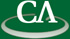## Calculating Tangent Cones With Triangular Decomposition.

### 12:30pm, Wednesday June 4th, K9509.

```Abstract:
Sometimes it is useful to investigate the local behaviour of a hypersurface
about a point. When this point is ‘nice’ (which in our case means
non-singular) the tangent plane provides an adequate linear description of
the space. However, when this point is singular, a bundle of tangens lines
is required.

This bundle is called the Tangent Cone and when working algebraically one
can calculate this by finding the homogeneous components of least degree
among the ideal the hypersurface generates. This is easy for principally
generated ideals, but for ideals with more than one generator Standard
bases are required which in turn require Groebner bases (GBs). As these
GBs are often prohibitively expensive to calculate there is utility in
finding a GB-free algorithm.

Indeed one can take an "algebraic limit" by finding secants modulo
triangular sets (in particular regular chains) utilizing efficient
splitting algorithms. We present an algorithm for computing a tangent cone
of a one-dimensional ideal at a point given by a zero-dimensional ideal
using Triangularization.

```